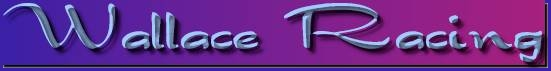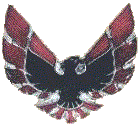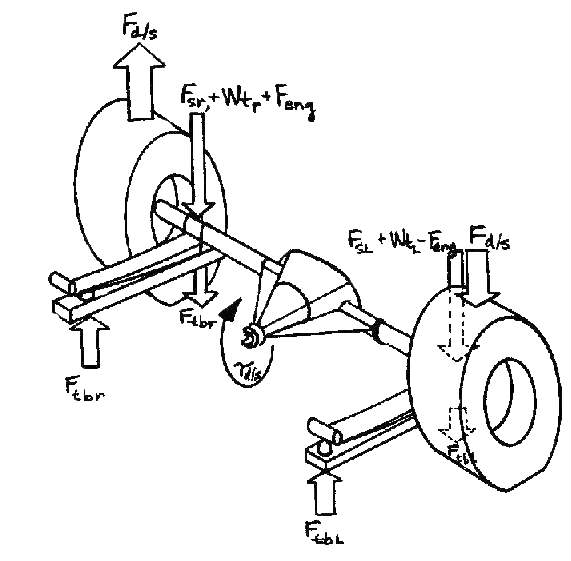PONTIAC POWER RULES ! ! !

PONTIAC POWER RULES ! ! !Leaf Spring TractionWallace RacingBACK to Tech Articles

This is taken from H-O Racing's High Output newsletter -Jan. 1975

TECHNICAL TOPIC: Drag Racing Leaf-Spring Rear Suspensions

Most racers have more performance potential in their Pontiac than they realize simply because they are not getting all their available power to the ground for maximum acceleration. Even though your drag racing Pontiac is leaving "straight" both rear tires may not be pulling with maximum tractive force or even equally: Therefore the subject of this Technical Topic is a simple analysis of the forces and torques acting on the rear suspension and how to compensate for the various unequal forces on a leaf-spring Pontiac such as a Firebird or Ventura II.

Below is a sketch of a typical leaf-spring suspension with traction bars. The forces acting on each rear wheel and the "upsetting" force due to the driveshaft torque are illustrated.

Forces on the right tire (passenger side) are:

Fr- Far + Wtr + Feng - Fd/s + Ftbr

Forces on the left tire (driver side) are:

Fl - Fsl + Wtl - Feng + Fd/s + FtblThe terms above are:

Fsr = force the right spring exerts on the axle housing (spring rate times deflection)

Fsl = same as Fsr, but for left side

Wtr = Weight on the right side due to uneven loaded weight (driver, etc.)

Wtl = same as Wtr, but for left side

Feng = Force on the chassis due to engine torque (engine torque divided by half the distance between spring perches)

Fd/s = Force on the rear axle due to driveshaft torque (engine torque times transmission gear ratio divided by half the distance between rear tires)

F tbr = Force exerted upon the chassis and down on the right side of the axle

by the traction bar (coefficient of tire friction times half the driveshaft torque times rear end gear ratio divided by the distance from the axle centerline to the traction bar snubber)

Ftbl= same as Ftbr but for the left side

The ideal situation is when the forces on both wheels are equal. To figure out how to get this ideal situations we have to know the contribution of each of the terms in the above formulas and adjust them accordingly. Let us look at an example and see how unevenly the tires are loaded in a typical situation. A 1973 Firebird Trans Am 455 Super Duty weighs about 3640. About 45% of the static weight is on the rear wheels so the force due to the rear springs (equal spring rates in this case) is 819 lb on each side. An average driver weight is 180 lb. About 60% of his weight is on the driver side and about 45% of that weight is on the rear wheels, so the uneven weight is 45 lb on the left side, 32 lb on the right side. A typical 455 SD engine torque value is 400 ft-lb.

The resultant chassis force due to engine torque is 213 1b (400 x 2/3.75). The resultant axle force due to driveshaft torque is 389 lb (400 x 2.43 x 2/5) . Adding everything up (with no traction bars considered) yields:

Force Left Side Right Side

Spring 819 lb 819 lb

Uneven weight 49         32

Engine Torque -213        213

Driveshaft torque 389       -389

Total 1044lb     675 lb

We see that without traction bars we are unevenly loaded by 369 lbs: If we are clever in our traction bar and tire adjustment, we can compensate for most of this. Referring back to the description of the force due to the traction bar, we note that both the coefficient of tire friction and the traction bar snubber distance are adjustable. Most traction bars such as JR Action Bars have provision for locating the snubber in one of two locations. The longer location (22") is used on the left side and the closer one (20") is used on the right side. If the coefficient of tire friction is 1.0, then the resultant left traction bar force is 1211 lbs (1 .0 x 400 x 2.43 x .5 x 4.56/1.83) and the resultant right traction bar force is 1327 lbs (1.0 x 400 x 2 .43 x .5 x 4.56/1.67) . The net difference is only ll6 lbs, so the Trans Am will still not be equally loaded but should run much quicker mostly because of the added tire loading provided by the traction bars (2538 lb total).

If we raise the pressure of the left side slick, it will reduce the coefficient of friction and the traction bar force. If we assume a coefficient of 0.79, the resultant left traction bar force will be 958 lb (instead of 1.211 lb) with a net difference of 369 lb compared to the right traction bar. This results in equal force on each tire, but unequal traction since the coefficient of friction is different for each tire since traction force equals coefficient of friction times tire loading force. If we lower the tire pressure in the left slick back towards its initial value to raise its coefficient of friction, we will eventually hit upon a coefficient value that results in equal traction. In this case the value is 0.925 for the left tire and 1.0 for the right tire. The resultant forces are:

Force                      Left Side           Right Side

Previous forces                 1044                 675

Traction Bar force             1120                1327

Total                           2164                 2002

Times coefficient of friction x0.925             x1.000

Traction force                  2002                2002

The Trans Am will be quicker yet, but still not at maximum potential since the left slick is working at less than maximum traction.

If we add spring force (stiffer spring, air lift bag, air shock, etc) to the right side and lower the left slick air pressure back to maximum traction value, the best traction situation will result. In this case, it takes 253 lb (1044 + 1211 - 2002) difference in spring force. Loading the right tire with spring force unloads the left one the same amount since total vehicle weight is unchanged. Therefore if we add 127 (253/2) spring force to the right side, the resultant traction forces are:

Force                 Left Side Right Side

Spring.                   692         946

Uneven weight              49          32

Engine torque            -213         213

Driveshaft torque         389        -389

Traction Bar            1211        1327

Total                    2128        2129

This set-up provides the maximum downward force on the tires (4257 lb) and although it is less than the maximum available acceleration force of 5540 lb (400 a 2.43 x 4.56 x 1.25), in actuality, chassis reaction due to the acceleration will provide additional spring force to increase the acceleration force and tend to keep the chassis level in the rear (keep the rear end from jumping up due to traction bar reaction). In any case, now you know how to go about getting that hole-shot on the competition!